Dark Energy and Estimate of Cosmological Constant from String Theory | OMICS International
Journal of Astrophysics & Aerospace Technology

# Dark Energy and Estimate of Cosmological Constant from String Theory

Rulin Xiu*

Hawaii Theoretical Physics Research Center, 16-266 E. Kipimana St, Keaau, HI 96749, USA

*Corresponding Author:
Rulin Xiu
Hawaii Theoretical Physics Research Center
16-266 E. Kipimana St, Keaau, HI 96749, USA
Tel: (415) 926-3196
E-mail: [email protected]

Received Date: March 17, 2017; Accepted Date: March 28, 2017; Published Date: March 31, 2017

Citation: Xiu R (2017) Dark Energy and Estimate of Cosmological Constant from String Theory. J Astrophys Aerospace Technol 5:141. doi: 10.4172/2329- 6542.1000141

Copyright: © 2017 Xiu R. This is an open-access article distributed under the terms of the Creative Commons Attribution License, which permits unrestricted use, distribution, and reproduction in any medium, provided the original author and source are credited.

Visit for more related articles at Journal of Astrophysics & Aerospace Technology

#### Abstract

The observation that expansion of our universe is accelerating suggests the existence of an unknown energy source, dark energy. The observed large-scale structure of our universe indicates the existence of an unknown matter source, dark matter. Cosmological constant, a constant term related to the vacuum energy appearing in the equation of gravitational field, is the simplest possible form of dark energy. String theory is one of the few mathematically consistent theories that can unify quantum physics and general relativity. In this work, we present a new way to derive and interpret string theory. We show that this new interpretation of string theory can explain the source of dark energy and dark matter. It may provide a way to calculate cosmological constant consistent with the current data.

#### Keywords

Cosmology; Dark energy; String theory; Universe

#### Introduction

In cosmology, cosmological constant is the value of vacuum energy density of space. It was originally introduced by Albert Einstein as an addition to his theory of general relativity to "hold back gravity" and achieve a static universe. The Hubble’s 1929 discovery that galaxies are moving away from each other implies an overall expanding universe. Studies and observation data in physics, astrophysics, and cosmology indicates that our universe starts from a big bang, followed almost instantaneously by cosmic inflation, an expansion of space from which the universe is thought to have emerged 13.799 billion years ago [1-3]. From 1929 until the early 1990s, most cosmology researchers assumed the cosmological constant to be zero.

Since the 1990s, several new discoveries in observational cosmology, especially the discovery of the accelerating universe from distant supernovae in 1998 in addition to independent evidence from the cosmic microwave background and large galaxy redshift surveys have shown that around 68.3% of the mass-energy density of the universe is due to dark energy [4-9] and around 26.8% is due to dark matter. Dark energy is an unknown energy source that is responsible for the accelerated expansion of the universe. Dark matter is an unknown matter source that is necessary to account for the observed large-scale structure in our universe. The cosmological constant is the simplest possible form of dark energy. Current standard model of cosmology, Lambda-CDM model, assumes non-zero cosmological constant as the source of dark energy. It provides a good fit to many cosmological observations as of 2016 with the cosmological constant to be on the order of 10−52 m−2 in metric units. In terms of Planck unit, and as a natural dimensionless value, the cosmological constant is calculated to be on the order of 10−121 .

The cosmological constant problem is the disagreement between the observed small value of cosmological constant and the theoretical non-zero vacuum energy as suggested by the quantum field theory. Depending on the assumptions, the discrepancy ranges from 40 to more than 100 orders of magnitude. Hobson and Efstathiou  describe this as "the worst theoretical prediction in the history of physics".

Some theorists resort to supersymmetric theories to solve the cosmological constant problem. Supersymmetry is the symmetry between the particles with integer spin (bosonic particles) and the particles with half spin (fermionic particles). Supersymmetric theories require a cosmological constant to be exactly zero. This does not solve the non-zero cosmological constant problem [11-13].

Another theoretical approach that attempts to solve the cosmological constant problem is multiverse theories [14,15]. Multiverse theory postulates a large number of "parallel" universes with different laws of physics and/or values of fundamental constants. One uses anthropic principle and prior probability distribution for cosmological constant over the multiverse to find the most probable value that allows galaxies to form. The anthropic principle is the philosophical consideration that observation of the universe must be compatible with the conscious and sapient life that observes it [16-18]. The argument is that only universes whose properties are such as to allow observers to exist are observed, while a possibly much larger set of universes lacking such properties go unnoticed.

In this paper, we will study dark energy, dark matter, and cosmological constant in the framework of universal wave function interpretation of string theory. As previously said string theory is a mathematically consistent theory that can incorporate both quantum theory and general relativity [11-13]. Quantum physics has been proven to be the fundamental physics for all elementary particles and three fundamental forces, electromagnetic, weak and strong forces. General Relativity is the proven theory for gravity. Many ways are proposed to incorporate gravity and general relativity into quantum physics. The grand unification theory, also called the theory of everything, is the attempt to use one mathematic formula to explain all the fundamental forces and matters discovered so far and to integrate quantum physics and general relativity together. String theory is a promising candidate for the grand unification theory [11-13].

How to understand and apply string theory has been a long debated subject. The currently accepted interpretation is that string theory studies the quantum dynamics of a string. The vibration of string creates particles and interactions. The scale of the string is set to be Planck Scale (approximately 1.6 × 10-35 meters). The finite size of the string length helps result in a consistent theory for all forces, including gravity. The achievement of string theory cannot be overestimated. This theory has the potential to predict the dimension of space-time, the particle and interaction spectrum, to unify all the forces and fundamental particles, and more. However, with all these great potentials and promises, string theory has not yet made many testable predictions. Something is still missing in our understanding of string theory.

In this work, using both quantum physics and general relativity, we obtain an uncertainty relationship (also called duality relationship) between space and time. From this relationship, we derive a new way to understand and apply string theory, the universal wave function interpretation of string theory (UWFIST). In quantum physics, everything is made of various waves and described by wave function. Wave function reveals the quality and quantities of the waves inside a system. We will show that our universal wave function interpretation of string theory provides a way to calculate the wave function of our universe. From the wave function of our universe, we can calculate the vacuum energy of our universe. We will demonstrate that using the age of our universe and fundamental constants such as the gravitational constant G, Planck constant Ä§, and speed of light, UWFIST provides a new way to understand the dark energy. It may yield the estimate of cosmological constant consistent with the current experimental observation.

#### A New Way to Derive and Interpret String Theory

Quantum physics has completely changed our views about spacetime in a way even more dramatic than the way Einstein has changed our view about space-time. According to quantum physics, how accurate we can measure time and space depends on the amount of energy and momentum we use. It takes energy and momentum to measure time and space. More specifically, according to quantum physics, to measure the time of duration Δτ, it takes the energy ΔE ~ Ä§/Δτ. Similarly, to measure space of size Δσ, it takes the momentum ΔP ~ Ä§/Δσ. Here Ä§ is the reduced Planck Constant, Ä§ = h/2π. And h is the Planck Constant. It is equal to 6.626 × 10−34 joul-second.

If one takes gravity into consideration, when one measures time interval Δτ, the energy ΔE used for time measurement will curve the space-time according to general relativity. It will create a black hole with the horizon on the order of GΔE/c4. Here G is the gravitational constant with the value approximately 6.674×10−11 N-m2/kg2 and c is the speed of light with the value approximately 3 × 108 meter/second. When Δσ is smaller than GΔE/c4, no information can come out. Therefore, the measurable causal region is: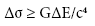From this, we find the condition for the causal region when we take both quantum effect and gravity into consideration is: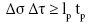(1)

Here lp is the Planck length, lp = (Ä§G/c3)1/2 = 1.616 × 10-35 meter. And tp is the Planck time, tp = (Ä§G/c5)1/2 = 5.39 × 10-44 second. This condition indicates an uncertainty relation between space Δσ and time Δτ in causal region. This relation is sometimes called duality relation. We suggest that this relation indicates that space and time are quantized and the non-commutation relation between space and time: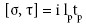(2)

Using the quantization procedure in quantum physics , we propose that the space time uncertainty relation (1) and noncommutation relation (2) indicates an action As in the form: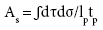(3)

Action As evolves the wave function from ψ (0,0) at the space 0 and time 0 to ψ (L,T) at the space L and time T in the following way: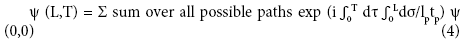It is interesting to note that action (3) is a string action [11-13]. This demonstrates from another angle that when we take gravity into consideration, it is necessary to use a “string” action (3) instead of “particle” action

A = Ê� dτ to obtain a consistent theory. We introduced the universal wave function ψ (L,T) in equation (4). We call equation (4) the universal wave function interpretation of string theory (UWFIST).

UWFIST deviates from the usual interpretation of string theory in two ways. Firstly, the world-sheet space σ and time τ integration of the action (3) is over the causal region. i.e.,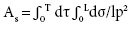Here T and L are the age and length of the causal horizon. In the normal interpretation of string theory, σ is the string coordinate. L is taken to be the length of the string. It is set to be Planck length lp. In the formula (4), σ and τ represent proper space and time. T and L are now variables. They change as universe evolves or as space and time pass by. The second difference between the usual string theory and UWFIST is that the universal wave function Ψ (L,T) are introduced. Here Ψ (0,0) and Ψ (L,T) represents the universal wave function at the initial space and time and at the space L and time T. We propose that the universal wave function could be the wave function of our universe. This is because if we extend the string action to the cases of superstring and heterotic string with background fields, the universal wave function Ψ can include all fundamental particles; all gauge interactions, gravity, and more. It also includes the large structures of our universe up to the scale of horizon. In other words, UWFIST could be the wave function of our universe. Except for these two major conceptual differences, the usual string theory calculation can still be applied in UWFIST.\

In the string theory, there are two sets of space-time: the 2-dimensional world-sheet (σ, τ) and the observed space-time Xμ (σ, τ). The observed space-time Xμ (σ,τ) is a projection from the world sheet to possibly a higher dimensional space-time. In terms of Xμ (σ,τ), the action As in (3) becomes: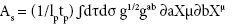(5)

Here gαβ is the metric tensor on the world-sheet formed by proper space and time (τ, ) and g = -det gαβ. As shown in string theory (Ref 1,2), the general form of action (5) in the presence of massless background field is in the form: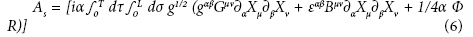Here Gμν (τ, σ), Bμν (τ, σ), Φ (τ, σ) are metric tensor, anti-symmetric tensor, and scalar background fields of the observed space. And R is the scalar curvature related to gαβ. The universal wave function in the presence of background fields is: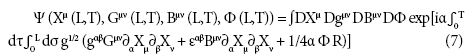We can extend Ψ (Xμ (L,T), Gμν (L,T), Bμν (L,T), Φ (L,T) to include other massive vibrations. We can also extend the above string action to the cases of superstring and heterotic string to include all fundamental particles, all gauge interactions, gravity, and more. This universal wave function tells us the probability for our universe to be at spacetime coordinate Xμ (L,T) and background fields Gμν (L,T), Bμν (L,T), Φ (L,T). We can do a Fourier transformation to transform the wave function Ψ (Xμ (L,T), Gμν (L,T), Bμν (L,T), Φ (L,T) to be expressed in the vibrational space expressed by the various vibrations (ωμμν,…). The wave function Ψ (ωμ (L,T),κμν (L,T),…) expresses what kind of vibrations in our universe and how much they exist at proper time T and horizon length L in our universe.

#### Existence of Dark Energy and Dark Matter

A natural indication and consequence of UWFIST is the existence of dark energy and dark matter. The space-time coordinate Xμ (τ, σ) is composed of vibrations in the form exp [iπn (σ+cτ)/L] and exp [iπn (σ-cτ)/L]. Here n is an integer. Unlike the normal string theory, in which L is the Planck length, now L is causal horizon distance. The largest wavelength is on the order of the length of the causal horizon. These vibrations are very fine. They are very dark in the sense that it is very difficult to detect them. In fact, to detect the vibration with the wavelength L, it takes the time L/c. If L is the horizon distance, this means that it takes the time of the age of the visible universe or a detector as large as the whole visible universe to detect these vibrations. The existence of these vibrations may explain the existence of dark energy.

There also exist vibrations with small masses, which can be as small as h/(Lc). These are extremely small masses. The existence of these vibrations with extremely small masses may explain the existence of dark matter. Similar to the vibrations that cause dark energy, it is very difficult, if not impossible, to directly detect these massive vibrations.

#### An Estimate of Vacuum Energy and Cosmological Constant

Now we are going to calculate the vacuum energy density in our observed space-time in the framework of UWFIST. Here we define the vacuum energy to be the lowest energy state of the universal wave function. Because our observed universe is the projection from the world-sheet, the world sheet contains all the information, energy, and matter about our universe. All the vibrations in the observed space time come from the vibrations in the world-sheet. Therefore, we simply need to calculate the vacuum energy in the two-dimensional world sheet.

To calculate the vacuum energy of the world-sheet, let’s assume that on the world-sheet, the energy is in the form of harmonic oscillator. The energy at the state (n,m) with the frequency νn = n/T and wavelength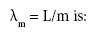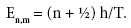The lowest energy of this vacuum fluctuation is E0n,m = h/2T.

The number of possible vibrations on the world sheet is TL/ (tplp). We can get this from the uncertainty relation (1). To make the space measurement Δσ, we should at least use the vibration with the wavelength λm = L/m ~ Δσ. To measure the time measurement Δτ, we should at least use the vibration with the frequency νn = n/T ~ Δτ. Since Δσ Δτ ≥ lp tp, we get nm < TL/(tplp).

The total lowest vacuum energy is: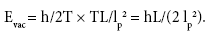(8)

It is proportional to the length of the causal horizon. The lowest vacuum energy density in the three dimensional space is: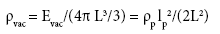(9)

Here ρp is the Planck energy density,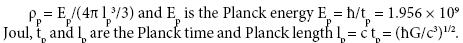The equation (5) tells us that the vacuum energy density observed in three-dimensional space is inversely proportional to the square of the length of horizon L.

The cosmological constant is defined as: Λc = 8π ρvac.

Therefore,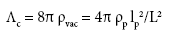We know that: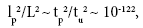Here tu is the age of the universe. We use:

tu =13.799 billion years = 4.35 × 1017 second.

From this, we obtain:Therefore within the UWFIST framework, using the age of the universe and the fundamental constants Ä§, G, and c (speed of light), we are able to estimate the value of cosmological constant to the same order of magnitude as the observed value.

#### Discussion

In this paper, we propose a new way to derive and understand string theory, the universal wave function formulation of string theory (UWFIST). From the space-time uncertainty relation, we show that string theory can be interpreted as calculating the evolution of universal wave function by the string action. We demonstrate that this new development of string theory can explain the source and existence of dark energy and dark matter. It can lead to the estimate of cosmological constant, possibly consistent with the experimental result using the age of the universe and the fundamental constants Ä§, G, and c.

#### Conclusion

This work gives a new scheme for cosmology. It provides a new way to understand and calculate dark energy and dark matter and to solve the cosmological constant problem from the fundamental physics theory. The detailed calculation of dark energy, dark matter, and cosmological constant is necessary to make the final conclusion about whether it can yield a plausible model for our universe. Further study and detailed calculations is needed to corroborate the result presented here.

#### Acknowledgements

This work is inspired by Dr. Zhigang Sha. I also want to thank Dr. Zhigang Sha, professor Mary K Gaillard, Dr. Ervin Laszlo, and many others for their help, support and encouragement with this project. I want to thank Rasha Blais, Alan Chuck, Marsha Valutis, and Christine Falcon for editing this paper.

#### References

Select your language of interest to view the total content in your interested language

### Article Usage

• Total views: 2665
• [From(publication date):
June-2017 - Dec 05, 2019]
• Breakdown by view type
• HTML page views : 2531Can't read the image? click here to refresh